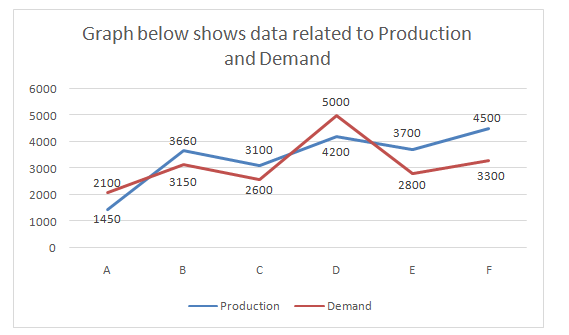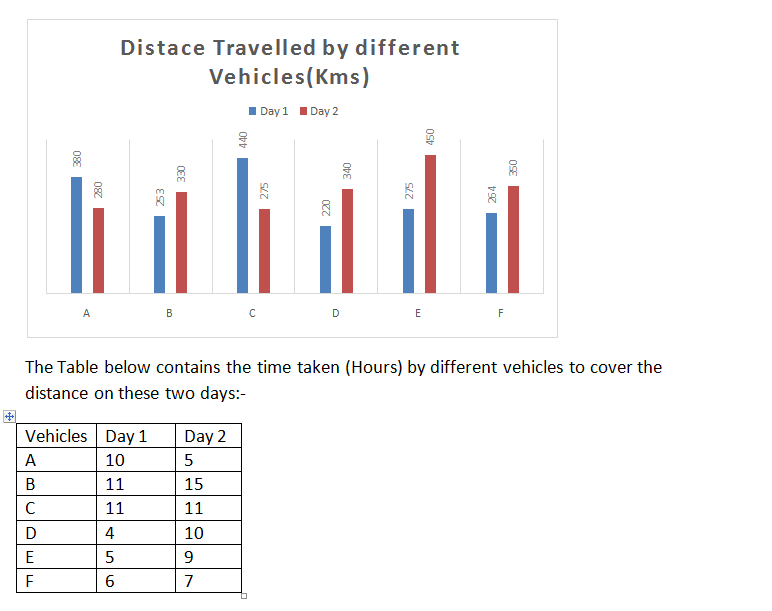# Quantitative Aptitude Questions (Data Interpretation) for SBI Clerk 2018 Day-16

## Quantitative Aptitude Questions (Data Interpretation) for SBI Clerk 2018 Day-16:

Dear Readers, SBI is conducting Online preliminary Examination for the recruitment of Clerical Cadre. preliminary Examination of SBI Clerk was scheduled from March 2018. To enrich your preparation here we have providing new series of Data Interpretation – Quantitative Aptitude Questions. Candidates those who are appearing in SBI Clerk Prelims Exam can practice these Quantitative Aptitude average questions daily and make your preparation effective.

Click “Start Quiz” to attend these Questions and view Solutions

## Daily Practice Test Schedule | Good Luck

 Topic Daily Publishing Time Daily News Papers & Editorials 8.00 AM Current Affairs Quiz 9.00 AM Logical Reasoning 10.00 AM Quantitative Aptitude “20-20” 11.00 AM Vocabulary (Based on The Hindu) 12.00 PM Static GK Quiz 1.00 PM English Language “20-20” 2.00 PM Banking Awareness Quiz 3.00 PM Reasoning Puzzles & Seating 4.00 PM Daily Current Affairs Updates 5.00 PM Data Interpretation / Application Sums (Topic Wise) 6.00 PM Reasoning Ability “20-20” 7.00 PM English Language (New Pattern Questions) 8.00 PM General / Financial Awareness Quiz 9.00 PM

Directions (Q. 1-5) Read the following graph carefully and answer the given questions:1. What is the ratio of no of companies having more demand while compared with production to those companies having more production while compared with demand?
1. 1:2
2. 2:3
3. 2:1
4. 3:2
5. None of these
1. What is the difference between the average demand and the average production of all the companies(approx)?
1. 450
2. 280
3. 277
4. 128
5. None of these
1. The difference between production and demand of company B is what percent more/ less than the difference between the production and demand of company F?
1. 57.5% less
2. 67.5% more
3. 50% more
4. 50% less
5. None of these
1. The production of company A is approximately what percent of the demand of company C?
1. 45
2. 74
3. 55
4. 23
5. None of these
1. The average production of companies C and D is approximately what percent of the average demand of A and E?
1. 149
2. 165
3. 76
4. 130
5. None of these

Directions (Q. 6-10) Study the following bar graph carefully and answer the given questions:1. The speed of Vehicle E on Day 2 is how much percent more or less than the speed of the Vehicle A on Day 1?( Approx)
1. 40
2. 23
3. 15
4. 32
5. None of these
1. The speed of vehicle F on day 3 is 20% more than its speed on day 2 then find the time taken by the vehicle on day 3 to travel 70 km more distance than on day 2?
1. 10
2. 12
3. 7
4. 17
5. None of these
1. What is the ratio between the total speed of all the vehicles on day 1 and day 2?
1. 85:79
2. 23:56
3. 67:49
4. 57:79
5. None of these
1. Vehicle B started its journey on day 3 with the same speed to travel the same distance as on day 2 but due to some breakdown it took 7 hours more than the usual time then find the difference between the speed on Day 1 and Day 3?
1. 18
2. 12
3. 8
4. 10
5. None of these
1. The distance travelled by vehicle B on day 2 is how much percent more than the distance travelled by vehicle D on Day 1?
1. 30
2. 25
3. 45
4. 50
5. None of these

Directions (Q. 1-5)

Companies A and D have more demand than production and the rest of them have more production than demand

Hence Required Ratio= 2:4= 1:2

The average demand= 2100+3150+2600+5000+2800+3300/6= 3158

The average production= 1450+3660+3100+4200+3700+4500/6= 3435

Required difference= 3435-3158= 277

Difference between production and demand of company B= 3660-3150= 510

Difference between production and demand of company F= 4500-3300= 1200

Required percentage= 690/1200*100= 57.5 % less

1450/2600*100= 55%

Average production C and D= (3100+4200)/2= 3650

Average demand of A and E= (2100+2800)/2= 2450

Required Percentage= 3650/2450*100= 148.97 %

Directions (Q. 6-10)

Speed of vehicle E on Day 2= 450/9= 50kmph

Speed of vehicle A on day 1= 380/10= 38kmph

Required percentage= 12/38*100= 32%

Speed of vehicle F on day 3= 120/100*50= 60kmph

Total distance on day 3= 350+70= 420 km

Required time= 420/60= 7 hours

Total speed of all vehicles on day 1= 38+23+40+55+55+44= 255

Total speed of all vehicles on day 2= 56+22+25+34+50+50= 237

Required ratio= 255:237= 85:79

Speed of vehicle B on day 3 = 330/22= 15 kmph

Speed of vehicle B on day 1= 253/11= 23 kmph

Required difference= 23-15= 8 kmph

Distance travelled by vehicle B on day 2= 330

Distance travelled by vehicle D on day 1= 220

Required percentage= (330-220)/220*100= 50%